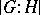# Subgroup, index of a

(diff) ← Older revision | Latest revision (diff) | Newer revision → (diff)
in a groupThe number of cosets (cf. Coset in a group) in any decomposition ofwith respect to this subgroup(in the infinite case, the cardinality of the set of these cosets). If the number of cosets is finite,is called a subgroup of finite index in. The intersection of a finite number of subgroups of finite index itself has finite index (Poincaré's theorem). The index of a subgroupinis usually denoted by. The product of the order of a subgroupby its indexis equal to the order of(Lagrange's theorem). This relationship applies to finite groupsand also to infinite groupsfor the corresponding cardinalities.